# Kerala Syllabus 8th Standard Basic Science Solutions Chapter 8 Measurements and Units

You can Download Measurements and Units Questions and Answers, Summary, Activity, Notes, Kerala Syllabus 8th Standard Basic Science Solutions Chapter 8 help you to revise complete Syllabus and score more marks in your examinations.

## Kerala State Syllabus 8th Standard Basic Science Solutions Chapter 8 Measurements and Units

In olden days different scales were used for measuring length in different countries. Vaara, One forearm (muzham) etc are some of such units. These units were not accurate. For solving this problem a standard scale of a definite length has been recognised world widw for measuring length. This standard scale is the unut of length. The basic unit of length is metre (m).

Smaller units of length

Examine the metre scale. You can see small measurements on it. These are centimetre (cm) and millimetre(mm)
1m = 1oocm 1cm=1omm
The smallest length that can be accurately measured using a metre scale is its least count.

Thickness of paper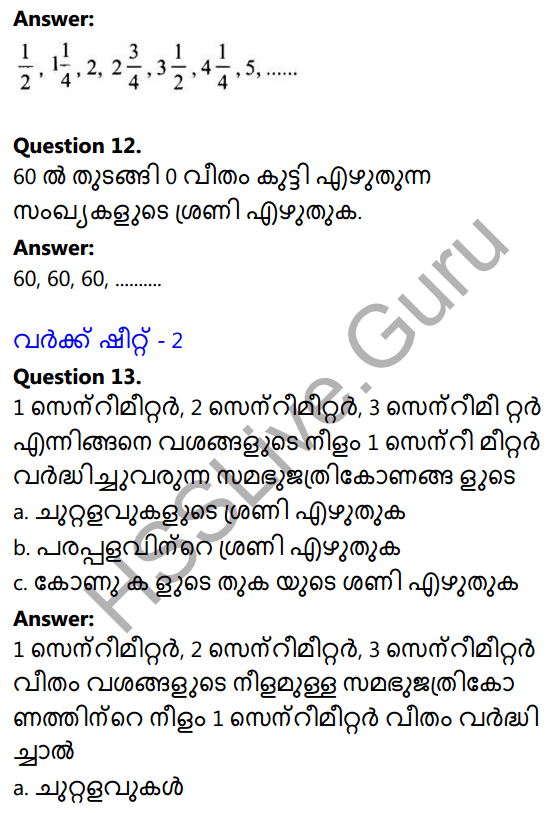We cannot measure the thickness of the paper even with these small units. Take too sheets of paper and pile it up to make a bundle. Measure the height of this bundle using a scale. If it falls in between two markings, make suitable changes in the number of sheets. Then measure its height. By dividing this height by the number of sheets, we will get the thickness of a sheet of paper.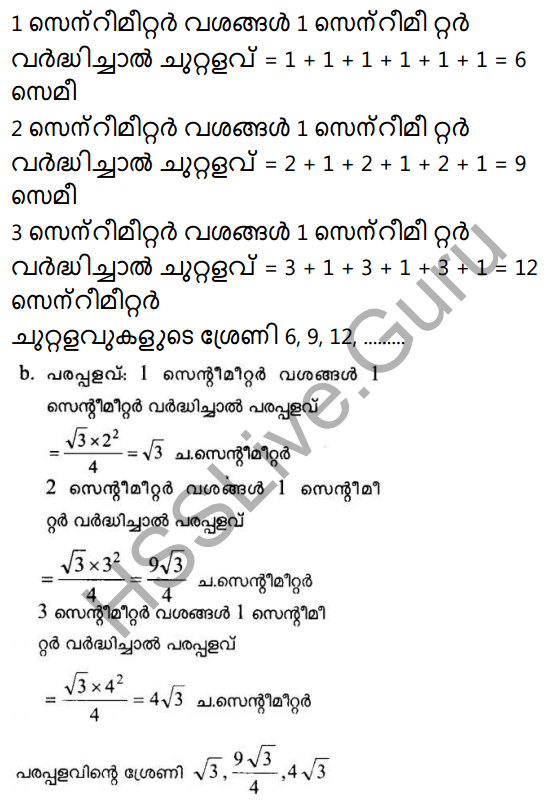Similarly to find out the thickness of thin wire roll it on a pencil and mea-sure the length of ten rolls Then divide it by 10.

Length of a curved line

To measure the length of the curved line put a thread on it and measure its length using a meter scale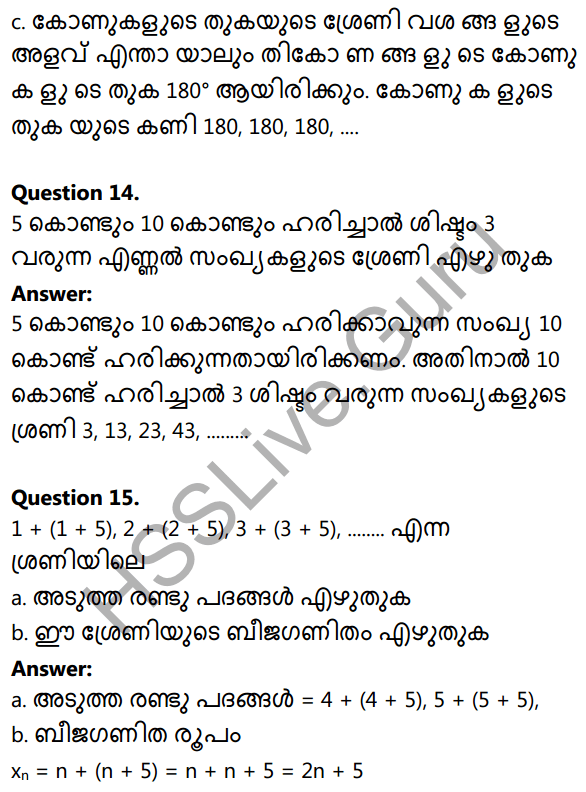Distance of a sphere

A ball kept in between two wooden blocks, whose diameter is to be measured. Then we will get its diameter.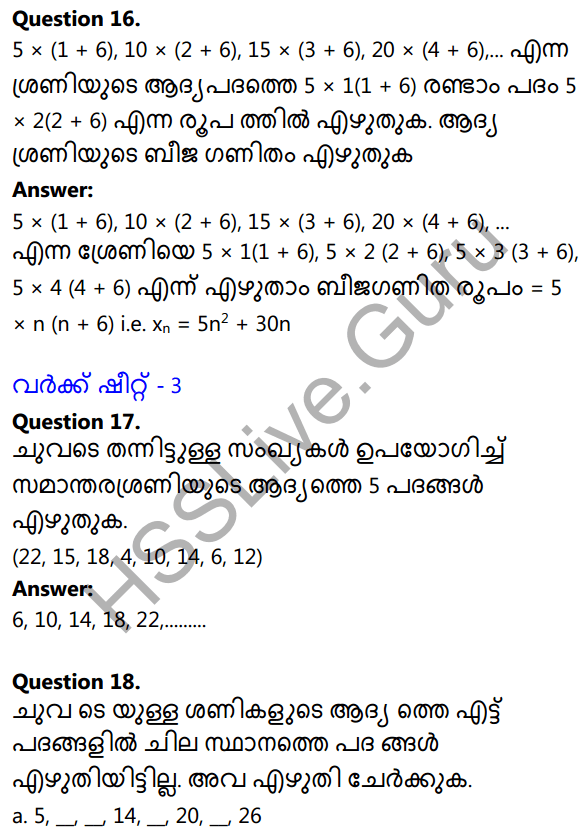Various measurements of length

The basic unit of length is metre. Smaller units like centimetre (cm), millimetre (mm), micrometre (micron – ™ nano-metre (nm) are also used in certain situa-tions for convenience. We use a bigger unit like kilometre (km) to measure the distance between two places. Units like astronomical unit (AU), light year (ly) are also used to measuring distance to planets or stars.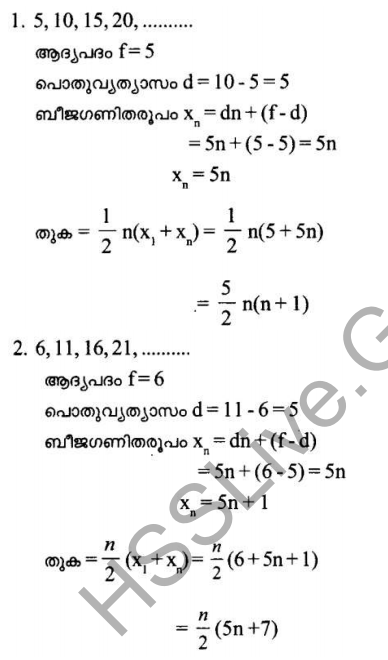Measurement And Units In Physics Class 8 Questions And Answers Mass

Mass is the measure of quantity of matter contained in it. The basic unit of mass is kilogram(kg).

Except kilogram, milligram(mg), gram(g), quintal, tonne are also the units of mass.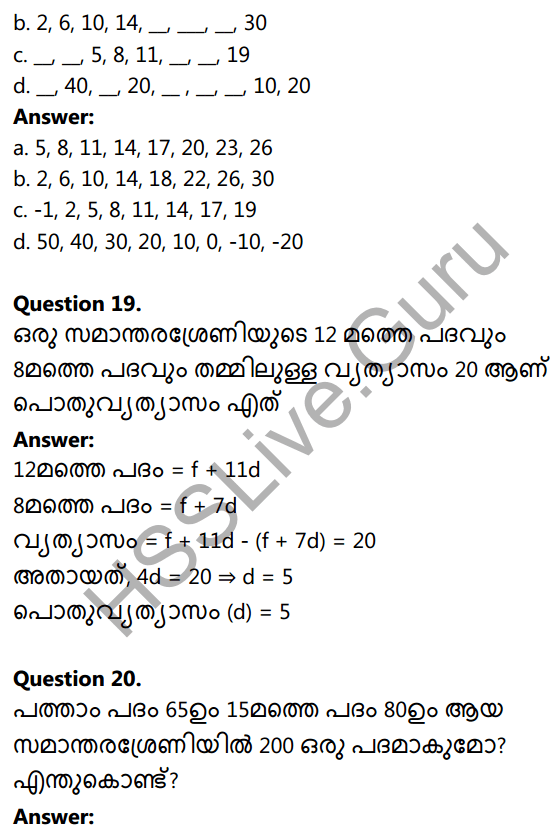Measurement And Units In Physics Class 8 Kerala Syllabus  Time
The symbol is s.
60s = iminute; 6ominute = 1 hour One second is 1/ 86400 part of an average solar day.

Fundamental units

There are some quantities which are not related to one another and cannot be expressed using other quantities. Such quantities are fundamental quantities. The units of the fundamental quantities are the Fundamental Units. The system based on these fundamental units is the | International System of Units. Its short form is SI Units.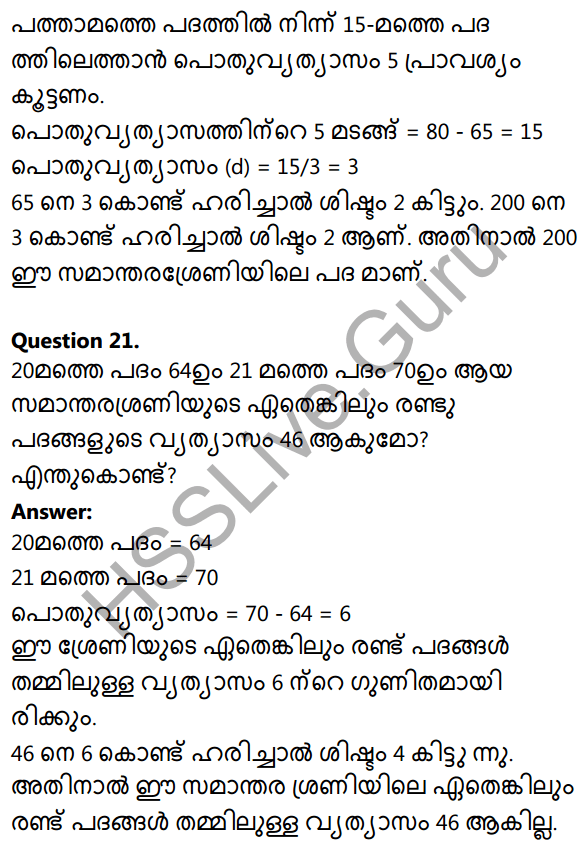Measurements And Units Class 8 Notes Kerala Syllabus Derived unit

Units which are expressed in terms of fundamental units or those units which are dependent on fundamental units are derived units.
Ex. Area = length x breadth

Unit of area = unit of length x unit of breadth = m xm = m2.Salient features of SI units

1. Unified units
2.  Internationally accepted
3.  Adequate to express all physical quantities.

Measurement And Units In Physics Class 8 Notes Density and volume

The space occupied by a body is referred to as its volume.
The unit of volume is cubic metre (m3)

Mass per unit volume of substance is its density. Density = mass/volume Unit of density = unit of mass/unit of volume = kg/m3

The rules to be followed while writing units

We have to observe certain rules according to international standards while using these units and their symbols. They are given below:

1. The symbols of units are normally written using small letters in the English alphabet, eg. m (metre), s (second), kg (kilogram)
2. But there are certain occasions on which capital letters of the English alphabet are used as symbols. The units named after persons are written like this.
3. While writing the names of units never use capital letters, eg. kelvin (correct) Kelvin (wrong) newton (correct) Newton (wrong)
4. Never use the plural form for symbols, eg. 10 kg (correct) 10 kgs (wrong) 75 cm (correct) 75 cms (wrong)
5. Never use full stop or comma after a
symbol except at the end of a sentence. eg. 75 cm is the length of a table, (correct) 75 cm. is the length of a table, (wrong),
6. While writing derived units a slash (/) is used to denote division. But never use more than one slash in one derived unit. eg. m/s1 (correct) m/s/ s (wrong)
7. When a derived unit is expressed as the product of other units use a dot or a space between them. eg. N.m or Nm
8. 8. Do not mix the name of a unit with the symbol.
9. While writing units along with a value, there must be single space between them.
10. Never use more than one unit to express a physical quantity.

### Measurements and Units Textbook Questions and Answers

Units And Measurements Class 8 Kerala Syllabus Question 1.
Write down the following units in the ascending order of their values
a) mm nm cm µ m.
b) 1 m 1 cm 1 km 1 mm
a. nm → µ m → mm → cm
b. 1 mm → 1 cm → 1 m → 1 km

Basic Science Question Answer Chapter Wise Class 8 Question 2.
Which of the following units does not belong to the group?
a) kg mg g mm
b) m mm km mg
a. mm
b. mgChemistry Textbook For Class 8 Kerala Syllabus Question 3.
Imagine that the distance from school to your friend’s house is 2250 m. State this in kilometres.
2$$\frac{1}{4}$$ km or 2.25 km

8th Class Physics Question And Answers Kerala Syllabus Question 4.
Convert the following units, into SI units without changing their values.
a. 3500 g
b. 2.5 km
c. 2 h
a. 3.5 kg
b. 2500 m
c. 7200 s

Kerala Syllabus 8th Standard Notes Physics Question 1.
Take a small quantity of solution A and solution B in a jar. Density of A is 2 kg/m3 and density of B is 5 kg/m3. Which of these flot above the jar ? Give reasion.
Solution A
Density of A is less than the density of B. So solution with less density floats above the solution that has high density.

Class 8 Chemistry Chapter 1 Kerala Syllabus Question 2.
Find out the given word pair relation; and fill in the blanks.
a. Length : m ; Volume :………
b. Displacement : m ; Acceleration:…………….
a. m3
b. m/s²Basic Science Class 8 Solutions Kerala Syllabus Question 3.
a. What is the basic unit of time?
b. What do you mean by one Solar day?
c. How much time earth will take to revolve once around the sun?
a. Second
b. A day or a solar day is the time period from one noon to the next noon.
c. One year (365 days)

Class 8 Science Notes Pdf State Syllabus Question 4.
a. meter, kilogram, kilogram/ meter3, second
b. Length, volume, mass, time
c. Volume, mass, density accelaration.
Ans:
a.kilogram/meter3 – meter, kilogram, second are basic units, kilogram/meter3 is unit of volume.
b. Volume – Volume is a derived quantity and others are basic quatities.
c. Mass – Mass is a basic quantity and others are derived quantity.

Class 8 Physics Kerala Syllabus Question 5.
What is meant by least count of a metre scale?
The smallest length that can be accurately measured using a metre scale is its least count. The least count is 1mm when metre scale is used.

Basic Science Class 8 Pdf Notes Kerala Syllabus Question 6.
Mention a method to measure the thickness of a paper sheet.
Take 100 sheets of paper and pile it up to make a bundle. Measure the height of this bundle using a scale. If it falls in between two markings, make suitable changes in the number of sheets. Then measure its height. By dividing this height by the number of sheets, we will get the thickness of a sheet of paper.

Hsslive Guru 8th Class Physics Kerala Syllabus Question 7.
Using following objects how can you measure the diameter of a ball?
hall, two wooden blocks, metre scale
The ball is kept in between two wooden blocks as shown in the figure whose diameter is to be measured.Basic Science Class 8 Kerala Syllabus Question 8.
Fill in the blanks
a. 1 nm = ….. m
b. 1 km = …….cm
c. 1000g = …….kg
d. one light year = …… km
e. 60 sec = …… minute
a. 1 nm = 10-9 m
b. 1 km = 105
c. 1000g = 1kg
d. one light year = 365 × 24 × 60 × 60 × 105 km
e. 6o sec = 1 minute

Chemistry Class 8 Kerala Syllabus Question 9.
Calculate how many seconds in a solar day.
24 ×60 × 60sStd 8 Biology Notes Malayalam Medium Kerala Syllabus Question 10.
Explain the derived units with examples.
Units which are expressed in terms of fundamental units or those units which are dependent on fundamen¬tal units are derived units.
Ex . Area = length × breadth=m × m = m2

Question 11.
Tabulate the fundamental quantities and their units.Question 12.
What are the salient features of SI units.?

• Unified units
• Internationally accepted
• Adequate to express all physical quantities.

Question 13.
Find the volume of a box having length 2m,breadth lm and height 0.5m
volume = length × breadth × height =2 × 1 × 0.5 = 1 m3

Question 14.
Will the mass be same if you take one thermocol and a wooden block of same volume?
No. Mass/Volume is density. The density of thermocol is lesser than that of wooden block. The mass will not be the same.

Question 15.
Take one jar with salt water and into both jars. In which jar the egg floated over the liquid. Justify your answer.
In the jar having salt water because it has more density.

Question 16.
Tabulate following units and symbols
a. Length
b.Mass
c. Potential difference
d. Force
e.Pressure
f. Time
g.Density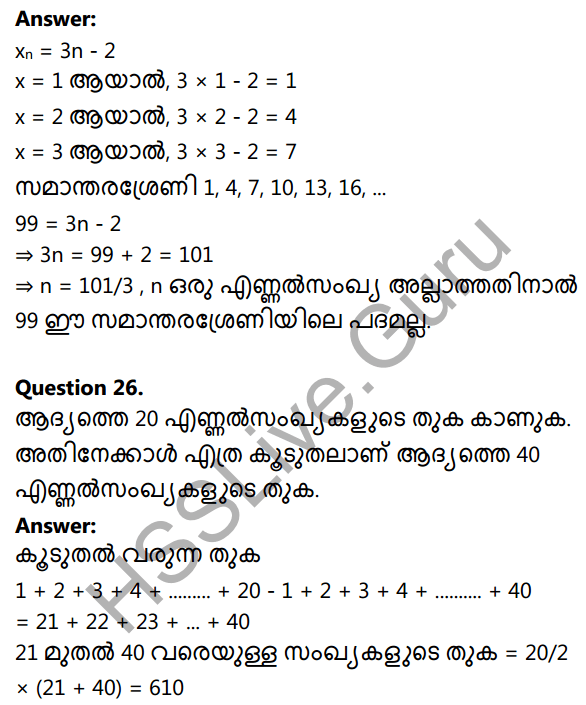Question 17.
Choose the derived quantities from following.
a. Length
b. volume
c. time
d. temperature
e. density
f. velocity
g. intensity of light
volume, density, velocity

Question 18.
Tabulate two units connected to the name of personalsQuestion 19.
Find the density of an object of mass 60 kg a having length 5m breadth 3m and height 2m.
density =mass ÷ volume
$$=\frac{60}{5 \times 3 \times 2}=\frac{60}{30}=2 \mathrm{kg} / \mathrm{m}^{3}$$

Question 20.
Find the volume of a brick which has a length, breadth and height of 3m, 2m and 4m respectively.
Volume = Length × breadth × height = 3 × 2 × 4 = 24m3

Question 21.
How to find the area of irregular surface? Explain with example.
The area of irregular surface can be calculated by using graph paper.

Question 22.
How do you find out the diameter of a torch cell
Place the cell between two wooden blocks tightly. Find out the distance between the two faces of the blocks, touching the cell using a metre scale. This reading gives the diameter of the cell.Question 23.
The length of a room is 8m and the breadth ia 5m. Calculate the area of the room.
Aiea = length × breadth = 8m × 5m = 40m2

Question 24.
What is meant by density of a substance?
Density is the mass of a substance having unit volume. If the mass of the substance is M and V is its volume,
then $$\frac{M}{V}$$

Question 25.
Find out the fundamental units from the following table and mark them. Find out which unit is used to measure each of the physical quantity.

• Ampere
• Metre/second
• Kilogram
• Kilogram/metre
• Mole
• Kelvin
• Metre3
• Kilogram metre/second